# Heron's Formula (Mathematics) Class 9 - NCERT Questions

Q 1.

A traffic signal board, indicating 'SCHOOL AHEAD', is an equilateral triangle with side 'a'. Find the area of the signal board, using Heron's formula. If its perimeter is 180 cm, what will be
the area of the signal board?

SOLUTION:

Each side of the triangle = a cm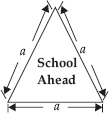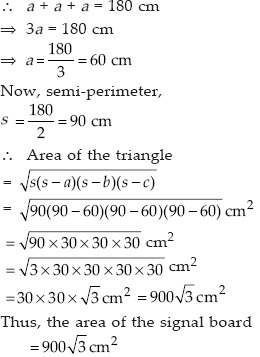Q 2.

The triangular side walls of a flyover have been used for advertisements. The sides of the walls are 122 m, 22 m and 120 m (see fig.). The advertisements yield an earning of ₹ 5000 per m² per
year. A company hired one of its walls for 3 months. How much rent did it pay?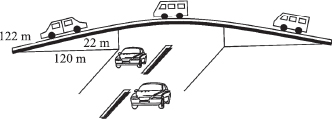SOLUTION:

The sides of the triangular wall are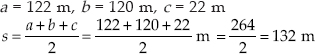The area of a triangular side wall is given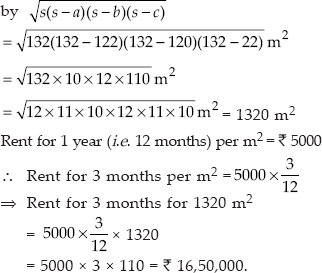Q 3.

There is a slide in a park. One of its side walls has been painted in some colour with a message 'KEEP THE PARK GREEN AND CLEAN' (see fig.). If the sides of the wall are 15 m, 11 m
and 6 m, find the area painted in colour.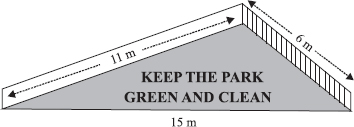SOLUTION:

Sides of the wall are 15 m, 11 m and 6 m.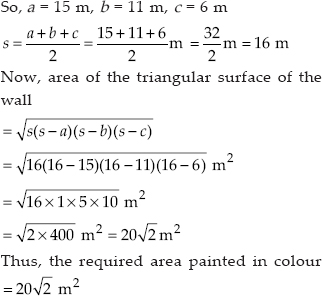Q 4.

Find the area of a triangle two sides of which are 18 cm and 10 cm and the perimeter is 42 cm.

SOLUTION:

Let the sides of the triangle be a = 18 cm, b = 10 cm and c = ?
Perimeter (2s) = 42 cm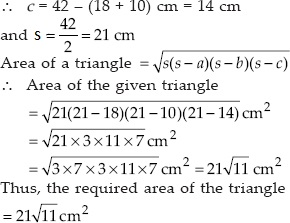Q 5.

Sides of a triangle are in the ratio of 12 : 17 : 25 and its perimeter is 540 cm. Find its area.

SOLUTION:

Perimeter of the triangle = 540 cm
⇒ Semi-perimeter of the triangle,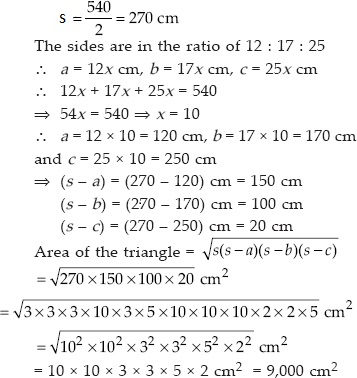Q 6.

An isosceles triangle has perimeter 30 cm and each of the equal sides is 12 cm. Find the area of the triangle.

SOLUTION:

Equal sides of the triangle are 12 cm each.
Let the third side = x cm.
Since, perimeter = 30 cm
i.e., 12 cm + 12 cm + x cm = 30 cm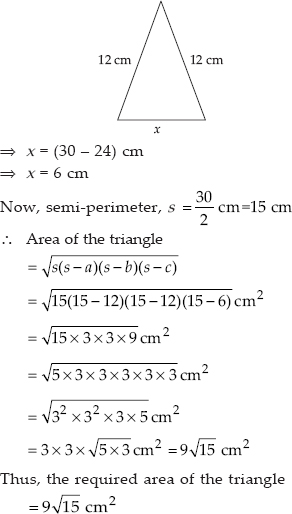Q 7.

A park, in the shape of a quadrilateral ABCD, has ∠C = 90°, AB = 9 m, BC = 12 m, CD = 5 m and AD = 8 m. How much area does it occupy?

SOLUTION:

Let us join B and D, such that ∆BCD is a right-angled triangle.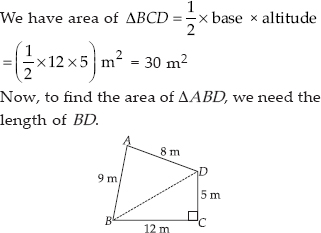In right angled ∠BCD,
BD² = BC² + CD² [Pythagoras theorem]
⇒ BD² = 12² + 5²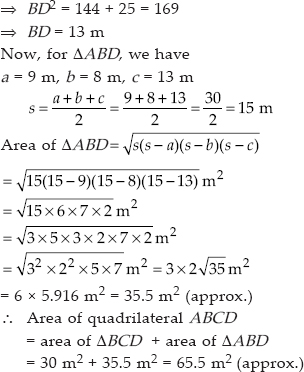Q 8.

Find the area of a quadrilateral ABCD in which AB = 3 cm, BC = 4 cm, CD = 4 cm, DA = 5 cm and AC = 5 cm.

SOLUTION:

For ∆ABC, a = 3 cm, b = 4 cm and c = 5 cm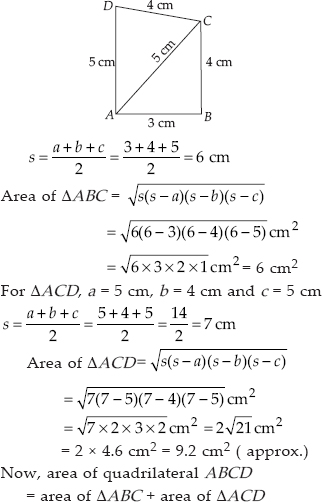= 6 cm² + 9.2 cm² = 15.2 cm² (approx.)

Q 9.

Radha made a picture of an aeroplane with coloured paper as shown in figure. Find the total area of the paper used.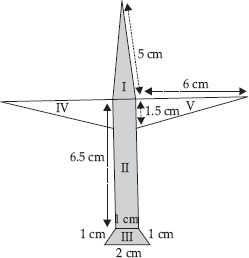SOLUTION:

Area of surface I :
It is an isosceles triangle whose sides are a = 5 cm, b = 5 cm, c = 1 cm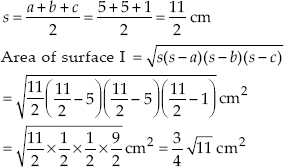= 0.75 x 3.3 cm²
= 2.475 cm² (approx.)
Area of surface II :
It is a rectangle with length 6.5 cm and breadth 1 cm.
Area of surface II = Length x Breadth
= 6.5 x 1 = 6.5 cm²
Area of surface III :
It is a trapezium whose parallel sides are 1 cm and 2 cm as shown in the adjoining figure.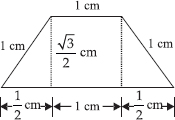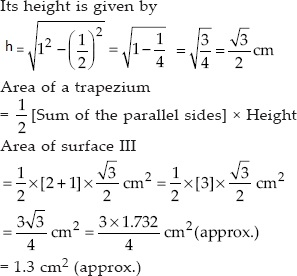Area of surface IV :
It is a right triangle with base as 6 cm and height as 1.5 cm.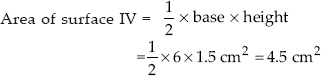Area of surface V
= Area of surface IV = 4.5 cm²
Thus, the total area of the paper used
= (area I) + (area II) + (area III) + (area IV) + (area V)
= [(2.475) + (6.5) + (1.3) + (4.5) + (4.5)] cm²
= 19.275 cm² = 19.3 cm² (approx.)

Q 10.

A triangle and a parallelogram have the same base and the same area. If the sides of the triangle are 26 cm, 28 cm and 30 cm, and the parallelogram stands on the base 28 cm, find the
height of the parallelogram.

SOLUTION:

For the given triangle, we have
a = 28 cm, b = 30 cm, c = 26 cm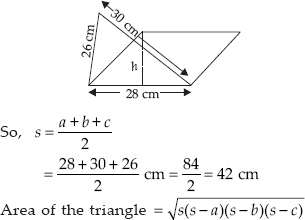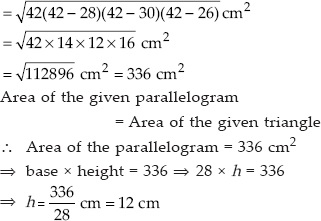Thus, the required height of the parallelogram = 12 cm

Q 11.

A rhombus shaped field has green grass for 18 cows to graze. If each side of the rhombus is 30 m and its longer diagonal is 48 m, how much area of grass field will each cow be getting

SOLUTION:

Here, each side of the rhombus = 30 m.
One of the diagonal = 48 m
Let ABCD be the given rhombus.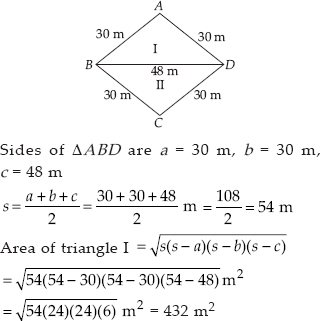Since a diagonal divides the rhombus into two congruent triangles.
Area of II triangle = 432 m²
⇒ The total area of the rhombus
= 432 m² + 432 m² = 864 m²
Area of grass for 18 cows = 864 m²
⇒ Area of grass for 1 cow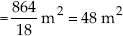Q 12.

An umbrella is made by stitching 10 triangular pieces of cloth of two different colours (see fig.), each piece measuring 20 cm, 50 cm and 50 cm. How much cloth of each colour is
required for the umbrella?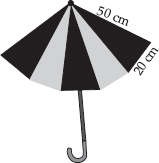SOLUTION:

Sides of each triangular piece are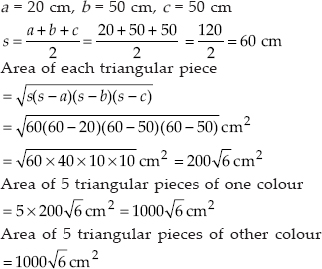Q 13.

A kite in the shape of a square with a diagonal 32 cm and an isosceles triangle of base 8 cm and sides 6 cm each is to be made of three different shades as shown in figure.
How much paper of each shade has been used in it?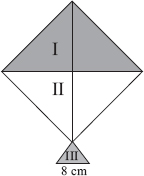SOLUTION:

Area of triangle I :
Diagonals of square are equal and bisect each other.
Therefore, AC = BD = 32 cm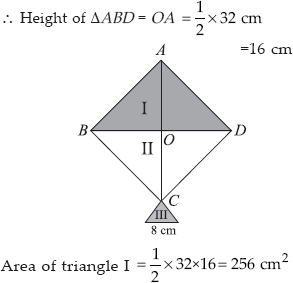Area of triangle II :
Since, diagonal of a square divides it into two congruent triangles.
So, area of triangle II = Area of triangle I
Therefore, Area of triangle II = 256 cm²
Area of triangle III :
The sides are given as a = 8 cm, b = 6 cm and c = 6 cm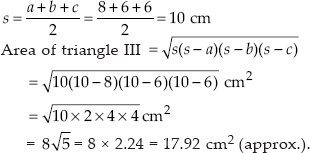Thus, the area of different shades are:
Area of shade I = 256 cm²,
Area of shade II = 256 cm²,
and area of shade III = 17.92 cm²

Q 14.

A floral design on a floor is made up of 16 tiles which are triangular, the sides of the triangle being 9 cm, 28 cm and 35 cm (see fig.). Find the cost of polishing the tiles at the rate of 50p per cm².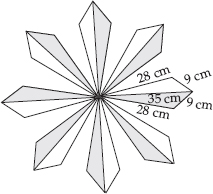SOLUTION:

Sides of the triangle are a = 9 cm, b = 28 cm, c = 35 cm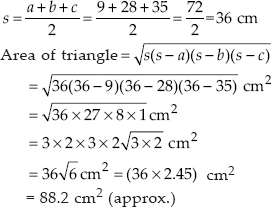Total area of all the 16 triangles
= (16 x 88.2) cm² = 1411.2 cm² (approx.)
Rate of polishing = ₹0.5 per cm²
Therefore, Cost of polishing all the tiles
= ₹ (0.5 x 1411.2) = ₹ 705.60 (approx.)

Q 15.

A field is in the shape of a trapezium whose parallel sides are 25 m and 10 m. The non-parallel sides are 14 m and 13 m. Find the area of the field.

SOLUTION:

Let the given field is in the form of a trapezium ABCD such that parallel sides are AB = 10m and DC = 25 m
Non-parallel sides are 13 m and 14 m.
We draw BE ∥ AD, such that BE = 13 m.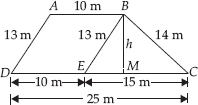The given field is divided into two shapes:
(i) parallelogram ABED
(ii) ∆BCE
Area of ∆BCE:
Sides of the triangle are a = 13 m, b = 14 m, c = 15 m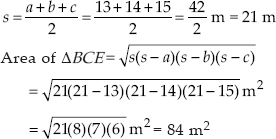Let the height of the ∆BCE corresponding to the side 15 m be h metres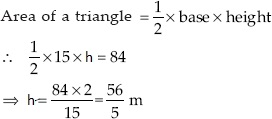Area of parallelogram ABED:
Area of a parallelogram = base x height
Therefore, Area of a parallelogram ABED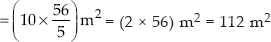So, area of the field
= area of ∆BCE + area of parallelogram ABED
= 84 m² + 112 m² = 196 m